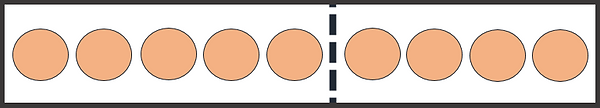top of page

## Topic 3: Odd and EvenSome numbers are odd, and some are even.

An even number can be shared to make two equal groups.

8 is even because it can be shared to make two groups of four:8 is even

(4+4)5 is odd

The odd and evens follow a pattern. They go odd, even, odd, even, and so on.

You should remember this pattern up until the number 9:1 is odd2 is even

(1+1)3 is odd4 is even

(2+2)5 is odd6 is even

(3+3)7 is odd8 is even

(4+4)9 is odd

This pattern carries on forever. Every odd number is followed by an even number, which is followed by another odd number.

For bigger numbers, you can tell if they are odd or even just by looking at the last digit. If the last digit is odd, the number is odd. If the last digit is even, the number is even.

So, 56 is even because its last digit is 6, which is even.

893 is odd, because its last digit is 3, which is odd.

Numbers that end in 0 (zero) are even. And zero itself is even. It is 0 + 0:0 is even

(0+0)

5 is odd because it cannot be shared this way. Whichever way you share 5, the groups are never equal:

bottom of page